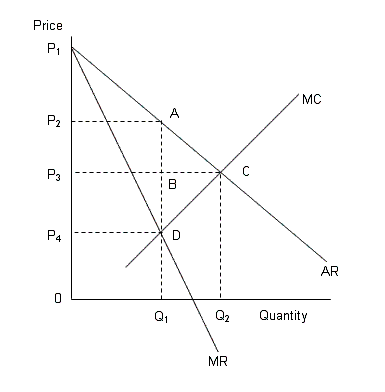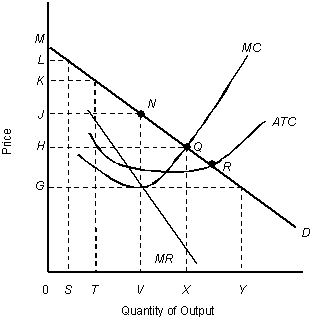/
/
/
81.Why does a efficiency loss arise under monopoly rather than
Not my Question
Flag Content

# Question : 81.Why does a efficiency loss arise under monopoly rather than : 1413826

81.Why does a efficiency loss arise under monopoly rather than under perfect competition?

a.A monopolist charges a lower price for the product to gain market entry.

b.A monopolist sells a lesser quantity at a higher price.

c.There is a net increase in consumer surplus but a net decline in producer surplus.

d.There is an increase in producer surplus, consumer surplus remaining unchanged.

e.A monopolist sells a greater quantity than a perfect competitor.

NARRBEGIN: Figure 10.8

The following figure shows revenue and cost curves of a monopolist.

Figure 10.8AR: Average revenue curve

MR: Marginal revenue curve

MC: Marginal cost curve

NARREND

82.According to Figure 10.7, the profit maximizing price of the monopolist is:

a.0.

b.P4.

c.P3.

d.P2.

e.P1.

83.According to Figure 10.7, what will be the price charged by a perfectly competitive firm?

a.P4

b.P3

c.P2

d.P1

e.0

84.According to Figure 10.7, the deadweight loss of monopoly is:

a.the area ABC.

b.the area BCD.

c.the area ACD.

d.the area P3P2AC.

e.the area P3P4DC.

NARRBEGIN: Figure 10.9

The figure given below shows the cost and revenue curves of a monopolist.

Figure 10.9D: Average revenue

MR: Marginal revenue

ATC: Average total cost

MC: Marginal cost

NARREND

85.In Figure 10.9, what is the consumer surplus at the profit-maximizing levels of output and price?

a.JNM

b.0JNV

c.HMQ

d.0HQX

e.JHQN

86.Refer to Figure 10.9. Suppose that the market is perfectly competitive. The consumer surplus would be represented by the area _____.

a.JNM

b.0JNV

c.HMQ

d.0HQX

e.JHQN

## Solution 5 (1 Ratings )

Solved
Economics 1 Year Ago 27 Views
This Question has Been Answered!